Next: Classical Interference of Light Up: Wave-Particle Duality Previous: Photoelectric Effect

# Quantum Theory of Light

According to Einstein's quantum theory of light, a monochromatic light wave of angular frequency, propagating through a vacuum, can be thought of as a stream of particles, called photons, of energy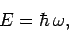(60)

where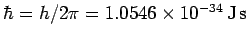. Since classical light waves propagate at the fixed velocity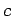, it stands to reason that photons must also move at this velocity. Now, according to Einstein's special theory of relativity, only massless particles can move at the speed of light in vacuum. Hence, photons must be massless. Special relativity also gives the following relationship between the energyand the momentumof a massless particle,(61)

Note that the above relation is consistent with Eq. (57), since if light is made up of a stream of photons, for which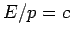, then the momentum density of light must be the energy density divided by. It follows from the previous two equations that photons carry momentum(62)

along their direction of motion, since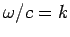for a light wave [see Eq. (50)].Next: Classical Interference of Light Up: Wave-Particle Duality Previous: Photoelectric Effect
Richard Fitzpatrick 2010-07-20